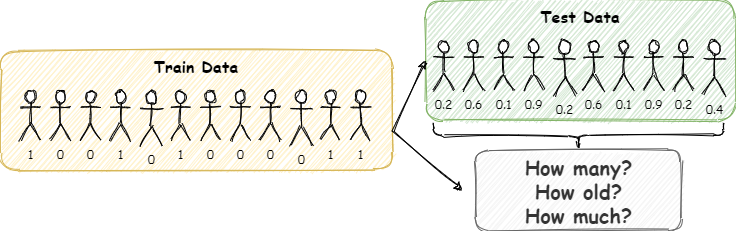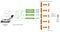1598792160

# How should we aggregate classification predictions?

If you are reading this, then you probably tried to predict who will survive the Titanic shipwreck. This Kaggle competition  is a canonical example of machine learning, and a right of passage for any aspiring data scientist. What if instead of predicting who  will survive, you only had to predict how many  will survive? Or, what if you had to predict the average age  of survivors, or the sum of fare  that the survivors paid?

There are many applications where classification predictions need to be aggregated. For example, a customer churn model may generate probabilities that a customer will churn but the business may be interested in how many customers are predicted to churn, or how much revenue will be lost. Similarly, a model may give a probability that a flight will be delayed but we may want to know how many flights will be delayed, or how many passengers are affected. Hong (2013) lists a number of other examples from actuarial assessment to warranty claims.

Most binary classification algorithms estimate probabilities that an example belongs to the positive class. If we treat these probabilities as known values (rather than estimates), then the number of positive cases is a random variable with Poisson Binomial probability distribution. (If the probabilities were all the same, the distribution would be Binomial.) Similarly, the sum of a two-value random variables where one value is a zero and the other value some other number (e.g. age, revenue) is distributed as Generalized Poisson Binomial. Under these assumptions we can report mean values as well as prediction intervals. In summary, if we had the true classification probabilities, then we could construct the probability distributions of any aggregate outcome (number of survivors, age, revenue, etc.).

Of course, the classification probabilities we obtain from machine learning models are just estimates. Therefore, treating the probabilities as known values may not be appropriate. (Essentially, we would be ignoring the sampling error in estimating these probabilities.) However, if we are interested only in the aggregate characteristics of survivors, perhaps we should focus on estimating parameters that describe the probability distributions of these aggregate characteristics. In other words, we should recognize that we have a numerical prediction problem rather than a classification problem.

In this note I compare two approaches to getting aggregate characteristics of Titanic survivors. The first is to classify and then aggregate. I estimate three popular classification models and then aggregate the resulting probabilities to get aggregate characteristics of survivors. The second approach is a regression model to estimate how aggregate characteristics of a group of passengers affect the share that survives. I evaluate each approach using many random splits of test and train data. The conclusion is that many classification models do poorly when the classification probabilities are aggregated.

#machine-learning #titanic #classification #aggregation #predictions

## Buddha Community1598792160

## How should we aggregate classification predictions?

If you are reading this, then you probably tried to predict who will survive the Titanic shipwreck. This Kaggle competition  is a canonical example of machine learning, and a right of passage for any aspiring data scientist. What if instead of predicting who  will survive, you only had to predict how many  will survive? Or, what if you had to predict the average age  of survivors, or the sum of fare  that the survivors paid?

There are many applications where classification predictions need to be aggregated. For example, a customer churn model may generate probabilities that a customer will churn but the business may be interested in how many customers are predicted to churn, or how much revenue will be lost. Similarly, a model may give a probability that a flight will be delayed but we may want to know how many flights will be delayed, or how many passengers are affected. Hong (2013) lists a number of other examples from actuarial assessment to warranty claims.

Most binary classification algorithms estimate probabilities that an example belongs to the positive class. If we treat these probabilities as known values (rather than estimates), then the number of positive cases is a random variable with Poisson Binomial probability distribution. (If the probabilities were all the same, the distribution would be Binomial.) Similarly, the sum of a two-value random variables where one value is a zero and the other value some other number (e.g. age, revenue) is distributed as Generalized Poisson Binomial. Under these assumptions we can report mean values as well as prediction intervals. In summary, if we had the true classification probabilities, then we could construct the probability distributions of any aggregate outcome (number of survivors, age, revenue, etc.).

Of course, the classification probabilities we obtain from machine learning models are just estimates. Therefore, treating the probabilities as known values may not be appropriate. (Essentially, we would be ignoring the sampling error in estimating these probabilities.) However, if we are interested only in the aggregate characteristics of survivors, perhaps we should focus on estimating parameters that describe the probability distributions of these aggregate characteristics. In other words, we should recognize that we have a numerical prediction problem rather than a classification problem.

In this note I compare two approaches to getting aggregate characteristics of Titanic survivors. The first is to classify and then aggregate. I estimate three popular classification models and then aggregate the resulting probabilities to get aggregate characteristics of survivors. The second approach is a regression model to estimate how aggregate characteristics of a group of passengers affect the share that survives. I evaluate each approach using many random splits of test and train data. The conclusion is that many classification models do poorly when the classification probabilities are aggregated.

#machine-learning #titanic #classification #aggregation #predictions1623223443

## Predictive Modeling in Data Science

#### Predictive modeling is an integral tool used in the data science world — learn the five primary predictive models and how to use them properly.

Predictive modeling in data science is used to answer the question “What is going to happen in the future, based on known past behaviors?” Modeling is an essential part of data science, and it is mainly divided into predictive and preventive modeling. Predictive modeling, also known as predictive analytics, is the process of using data and statistical algorithms to predict outcomes with data models. Anything from sports outcomes, television ratings to technological advances, and corporate economies can be predicted using these models.

### Top 5 Predictive Models

1. Classification Model: It is the simplest of all predictive analytics models. It puts data in categories based on its historical data. Classification models are best to answer “yes or no” types of questions.
2. Clustering Model: This model groups data points into separate groups, based on similar behavior.
3. **Forecast Model: **One of the most widely used predictive analytics models. It deals with metric value prediction, and this model can be applied wherever historical numerical data is available.
4. Outliers Model: This model, as the name suggests, is oriented around exceptional data entries within a dataset. It can identify exceptional figures either by themselves or in concurrence with other numbers and categories.
5. Time Series Model: This predictive model consists of a series of data points captured, using time as the input limit. It uses the data from previous years to develop a numerical metric and predicts the next three to six weeks of data using that metric.

#big data #data science #predictive analytics #predictive analysis #predictive modeling #predictive models1617419868

## Top Five Artificial Intelligence Predictions For 2021

As AI becomes more ubiquitous, it’s also become more autonomous — able to act on its own without human supervision. This demonstrates progress, but it also introduces concerns around control over AI. The AI Arms Race has driven organizations everywhere to deliver the most sophisticated algorithms around, but this can come at a price, ignoring cultural and ethical values that are critical to responsible AI. Here are five predictions on what we should expect to see in AI in 2021:

1. Something’s going to give around AI governance
2. Most consumers will continue to be sceptical of AI
3. Digital transformation (DX) finds its moment
4. Organizations will increasingly push AI to the edge
5. ModelOps will become the “go-to” approach for AI deployment.

#opinions #2021 ai predictions #ai predictions for 2021 #artificial intelligence predictions #five artificial intelligence predictions for 20211601269980

## Predict using classification methods in R

In this analysis i’ll build a model that will predict whether a tumor is malignant or benign, based on data from a study on breast cancer. Classification algorithms will be used in the modelling process.

The dataset

**The data for this analysis refer to 569 patients from a study on breast cancer. The actual data can be found at UCI (Machine Learning Repository): **https://archive.ics.uci.edu/ml/datasets/Breast+Cancer+Wisconsin+(Diagnostic). The variables were computed from a digitized image of a breast mass and describe characteristics of the cell nucleus present in the image. In particular the variables are the following:

1. **radius **(mean of distances from center to points on the perimeter)
2. **texture **(standard deviation of gray-scale values)
3. perimeter
4. area
5. **smoothness **(local variation in radius lengths)
6. **compactness **(perimeter^² / area — 1.0)
7. **concavity **(severity of concave portions of the contour)
8. **concave points **(number of concave portions of the contour)
9. symmetry
10. fractal dimension (“coastline approximation” — 1)
11. **type **(tumor can be either malignant -M- or benign -B-)

#predictive-analytics #logistic-regression #machine-learning #classification #decision-tree-classifier1601517600

## Feature pyramid network for image classification

Object detection is one of the main problems in computer vision that may fail when there are multi-scale objects in images. Using feature pyramids helps to solve this problem.

Some previous studies tried to use different kinds of feature pyramids to improve object detection. One method fed various sizes of the input image to the deep network to see objects with different scales. This way also helped improve object detection but increases computational costs and processing time so much that it is not efficient.

Feature pyramid network(FPN) was introduced by Tsung-Yi Lin et al., which enhanced object detection accuracy for deep convolutional object detectors. FPN solves this problem by generating a bottom-up and a top-down feature hierarchy with lateral connections from the network’s generated features at different scales. This helps the network generate more semantic features, so using FPN helps increase detection accuracy when there are objects with various scales in the image while not changing detection speed.

_Here, I aim to introduce a new architecture based on FPN to improve classification accuracy. This architecture is proposed in my _paper.

As described, FPN helps extract multi-scale features from the input image, which better presents objects with different scales. We have designed an architecture that utilizes FPN to understand better the important parts of the image that could exist in different sizes.

In the next figure, you can see our proposed architecture. This architecture was developed for classifying the patient CT scan images into normal and COVID-19. Researchers can modify this architecture for using on different datasets and classes.#image-classification #neural-networks #classification #deep-learning #machine-learning